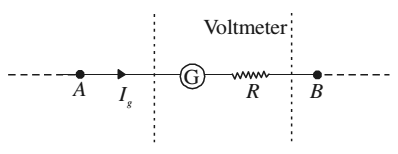# Voltmeter

A voltmeter is used to measure the potential difference between two points in a circuit. You can convert a galvanometer into a voltmeter by connecting a high resistance in series with the galvanometer coil.The value of the resistance depends on the range of voltmeter. A high resistance, say R is connected in series with the galvanometer coil. If the potential difference across AB is V volt, then total resistance of the voltmeter will be G + R. From Ohm’s law,

Ig(G + R) = V

G + R = v/Ig

R = V/Ig - G

If a resistance R is connected in series with the coil of the galvanometer, it works as a voltmeter of range 0-V volts.

The same scale of the galvanometer which was recording the maximum potential Ig×G before conversion will record the potential V after conversion into voltmeter. The scale can be calibrated accordingly. The resistance of the voltmeter is higher than the resistance of galvanometer. Effective resistance of the voltmeter, is given by

RV = R + G

The resistance of an ideal voltmeter is infinite. It is connected in parallel to the points across which potential drop is to be measured in a circuit. It will not draw any current. But the galvanometer coil deflects.